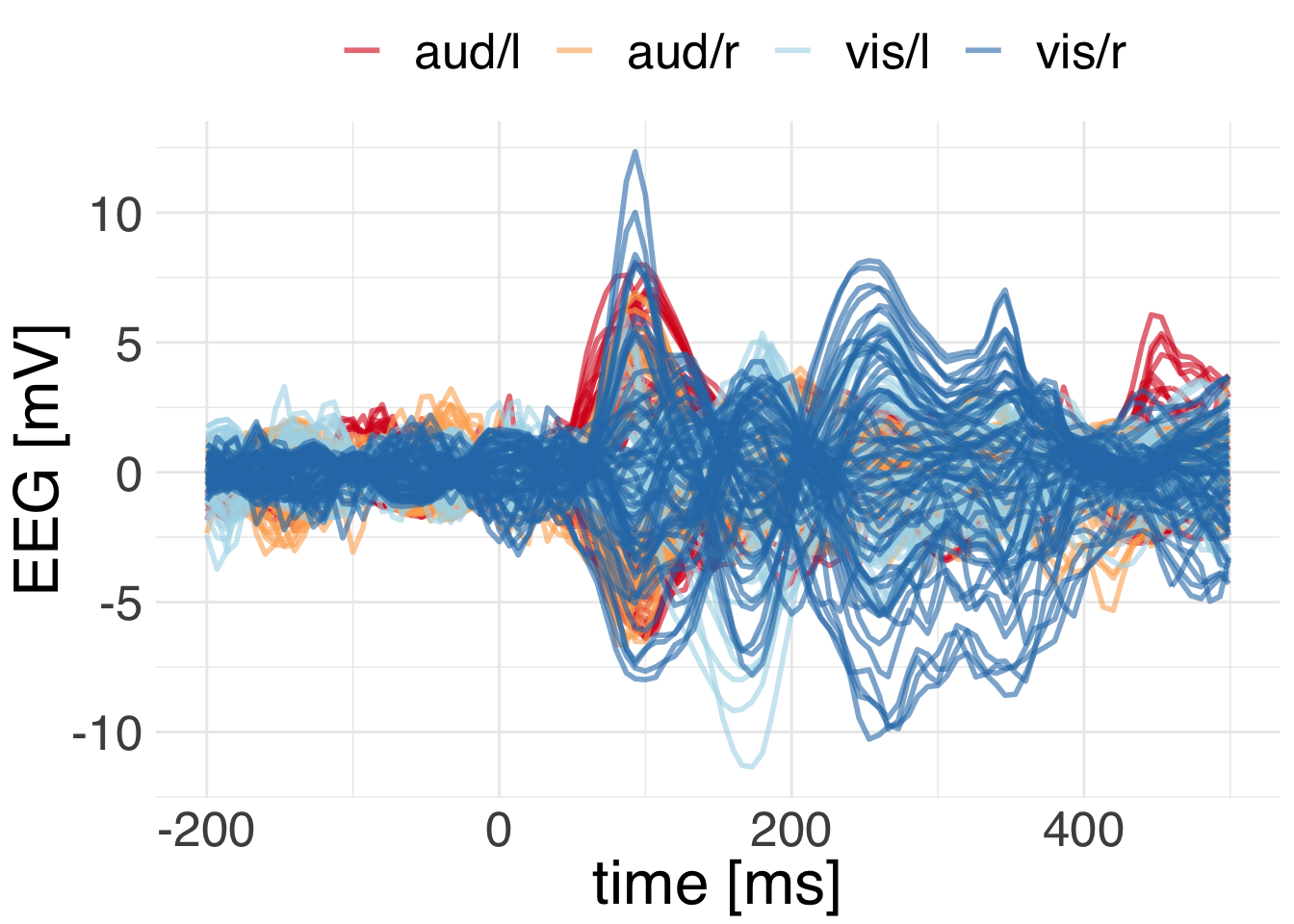In this vignette we will develop content that shows how to do averaging by hand in R.

library(tidyverse)
library(mne)
## Importing MNE version=0.18.dev0, path='/Users/dengeman/github/mne-python/mne'

Let’s read in the raw data.

data_path <- mne$datasets$sample$data_path() subject <- "sample" raw_fname <- paste(data_path, 'MEG', subject, 'sample_audvis_filt-0-40_raw.fif', sep = '/') raw <- mne$io$read_raw_fif(raw_fname, preload = T) raw$filter(1, 40)
## <Raw  |  sample_audvis_filt-0-40_raw.fif, n_channels x n_times : 376 x 41700 (277.7 sec), ~123.3 MB, data loaded>
events <- mne$find_events(raw) storage.mode(events) <- "integer" # R gets the events as floats. tmin <- -0.2 tmax <- 0.5 baseline <- reticulate::tuple(NULL, 0) event_id <- list("aud/l" = 1L, "aud/r" = 2L, "vis/l" = 3L, "vis/r" = 4L) picks <- mne$pick_types(raw$info, meg = T, eeg = T) epochs <- mne$Epochs(raw = raw, events = events, event_id =event_id,
tmin = tmin, tmax = tmax,
picks = picks %>% as.integer(),
baseline = baseline, reject = NULL, preload = T) 

We’ll get the epochs from mne and then average by hand.

# Let's get the epochs in wide format
epochs_df <- mne::get_data_frame(epochs, long_format = F)
## Deleting whitespace in channel names ...
# get rid of info variables: "condition", "epoch", "time"
ch_names <- colnames(epochs_df)[-c(1:3)]

# modify .funs to estimate the average differently.
evoked_df <- epochs_df %>%
group_by(time, condition) %>%
summarize_at(.funs = mean, .vars = ch_names) %>%
gather(key = "channel", value = "signal", -time, -condition)

Let’s plot the EEG channels.

ggplot(
data = evoked_df %>% filter(str_detect(channel, "EEG")),
mapping = aes(x = time, y = signal,
group = interaction(channel, condition),
color = condition)) +
geom_line(alpha = 0.6, size = 1) +
scale_color_brewer(palette="RdYlBu") +
theme_minimal() +
theme(text = element_text(size = 24, family = "Helvetica"),
legend.position = "top")+
labs(y = "EEG [mV]", x = "time [ms]", color = "")We can clearly see that the visual responses show more energy at greater latency than the auditory responses. Both peak around 100 milliseconds.